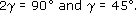Science, Maths & Technology

### Become an OU studentGeometry

Start this free course now. Just create an account and sign in. Enrol and complete the course for a free statement of participation or digital badge if available.

# Try some yourself

## Question 1

Find the unknown angles in each of these diagrams, which represent part of the bracing structure supporting a marquee.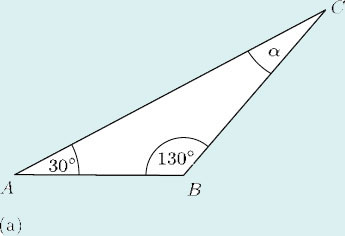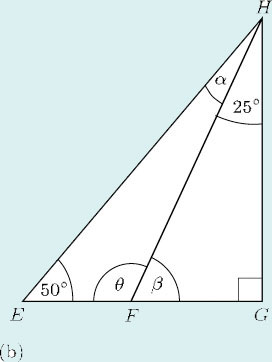• (a) In ΔABC,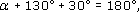therefore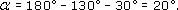• (b) In ΔFGH,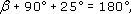therefore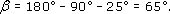As EFG is a straight line,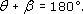So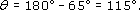In ΔEFH,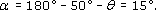## Question 2

Deduce the value of α in the triangle below.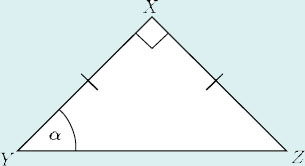Lines XY and XZ are of equal length. This means that the triangle is isosceles, so the base anglesand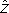are equal. Then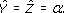The third angle in the triangle is a right angle: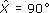.

Because the three angles in a triangle must add up to 180°,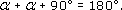Hence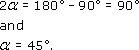## Question 3

Find the unknown angles in the following isosceles triangles, which represent roof rafters.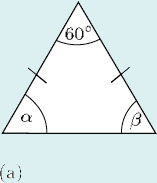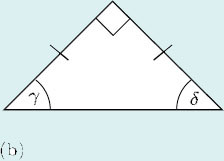• (a) As this is an isosceles triangle, α = β.

So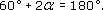Therefore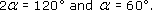• (b) As this is an isosceles triangle, γ = δ.

So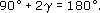Therefore